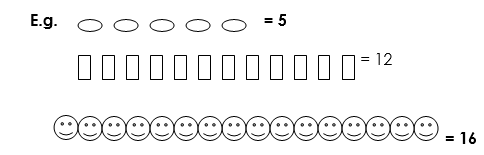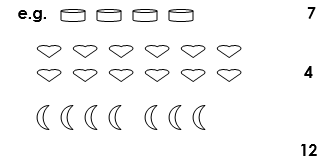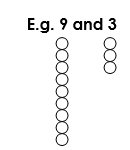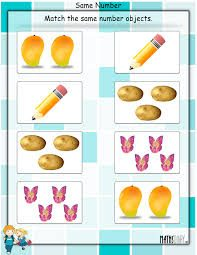# COUNTING,MATCHING AND COMPARISON OF OBJECTS-P.1

##### This unit is about counting,comparing and matching of objects

Lesson1

Counting objectsMatching pictures to numbers and vice versa.Activity: counting and matching (Prepared work on paper-refer to Mtc. File)

Comparing pairs of numbers up to 100

• using ‘greater than’ and ‘less than’ (bigger than/ smaller than)
• The greater number has more things and the less number has less or few things.Activity: counting and matching

Comparing pairs of numbers up to 100

• using ‘greater than’ and ‘less than’ (bigger than/ smaller than)
• The greater number has more things and the less number has less or few things.

e.g  9 and 3 – 9 is greater than/ bigger than 3.

–   3 is less than/ smaller than 9.

Activity: A: which number is greater?

1) 4 and 10 2) 13 and 18 3) 14 and 9 4) 15 and 20

5) 17 and 29 6) 30 and 40 7) 80 and 18 8) 11 and 5

B: Which number is less?

1) 10 and 20 2) 2 and 1 3) 5 and 8 4) 37 and 17

5) 18 and 28 6) 70 and 51 7) 3 and 27 8) 45 and 15

SEE ALL
•YOU